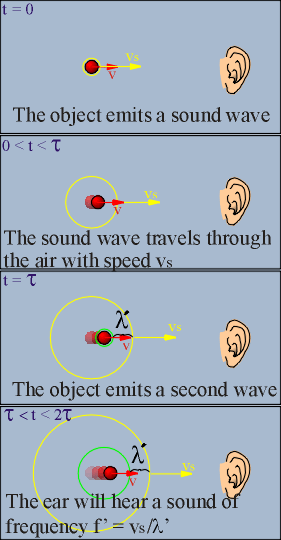### The sound of a moving object will change pitch if it is moving!

Watch the Video:

Teachable Topics:

• Speed of sound
• Frequency
• Wavelength

Theory:

As cars pass on the highway, or a train goes by on the tracks, do you ever notice how the pitch of the sound suddenly drops? This is due to the Doppler effect.

Consider a bell that rings with frequency f when at rest. It begins to move in the direction of a detector (your ear, perhaps) with a velocity, v.

Now imagine one crest of the sound wave the bell is producing. At time t = 0, the crest is emitted and moves through the air at vs, the speed of sound, regardless of the motion of the source. The time it takes for the next crest to be emitted is 1/f = τ (that is, the period of the sound). In this time, the first wave moves a distance vsτ towards the detector, and the source moves a distance vτ. The distance between the first and the second crest is then vsτ - v τ = (vs- v)τ. Because the distance between adjacent crests on a wave is the wavelength, λ', then λ' = (vs - v)τ.

Note that this wavelength, λ', is marked with a prime because it is not the wavelength that would be detected if the source were at rest - it is the shortened wavelength that is perceived by the detector. The perceived frequency is related to this perceived wavelength by the following formula:

λ' = vs/f

We derived above that λ' = (vs - v)τ

We know that τ = 1/f , so λ' = (vs- v)/f

We also know that λ' = vs/f', so vs/f' = (vs - v)/f. Rewritten, the formula says

f' = vs/(vs - v) f

This is the equation for the detected frequency if the source is approaching the detector. If the source is receding, then the same formula applies, but the velocity used has to be a negative number.

The Doppler effect can also be observed if the source is stationary and the detector moves, or if both source and detector move. Formulas for these situations are somewhat different from the one above, but are derived in a similar way.Figure 1: The Doppler Effect

Apparatus:

• A Doppler ball (i.e. a soft ball with a battery-operated buzzer inside)

Procedure:

• Turn on the Doppler ball to start the sound.
• In a large, open space, get the ball to travel in the direction of an observer.
• You can throw the ball over the observer's head, or, like we did, you can attach the ball to the helmet of a cyclist and have him drive by as quick as he can.